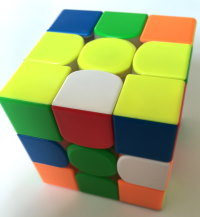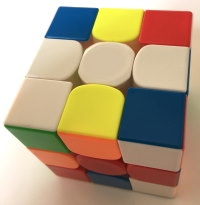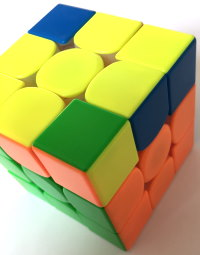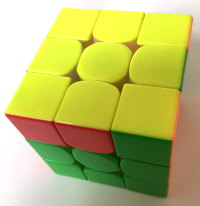# Ruwix ZZ Tutorial

Released in 2006, the ZZ method is a 3x3 speedsolving method developed by Zbigniew Zborowski. There are 4 different main variants, these being ZZ-a, the original, ZZ-b, ZZ-c, and ZZ-d. This tutorial will teach you the beginner ZZ method.

The steps of the beginner ZZ method are EOLine, F2L and LL.

[hide]
[show]
Contents

EOLine is the process of orienting all the edges on the cube so that it can then be solved RUL gen, while also building a line with the D face center and the DF and DB edges. F2L is solving the First 2 Layers, after which the cube should be a CFOP state where the U layer edges are always oriented, and then the last layer, which can be done in many ways.

Some well known cubers who use ZZ include Zbigniew Zborowski, the creator, and Phil Yu, aka asmallkitten, who started the famous cube store TheCubicle. ZZ tends to be classified as one of the Big Four Methods, which are CFOP, Roux, ZZ, and Petrus (although Petrus is occasionally omitted).

## EOLineThis step, at least for beginners, is usually split into two sub-steps: the EO (edge orientation) and the Line.

For the purposes of this tutorial, white will be held on the bottom, yellow on the top, and green facing you (assuming you have the normal color scheme). A good edge is an edge that can be solved with only RUL moves, whereas a bad edge for this purpose is an edge that needs F/B/D/E/M/S moves to solve.

All of the edges have a “key sticker”. There are 12 edges on the cube, 4 of which have a white sticker on them, 4 of which have a yellow sticker, and 4 that have neither. The key stickers are: for any edges that have a white sticker, the white sticker is the key one, for only edges that have a yellow sticker, the yellow is the key one, and for the remaining 4 the blue and green stickers would be the key ones. If you are solving with a color other than white on the bottom, just follow the rule that the top and bottom sides are the key colors, and the front and back sides also.To start, look at the U and D faces. If you see red or orange (or whatever color you have on the left or right) then that edge is bad. If you see green or blue (or whatever color you have on the front or back), then look at the other sticker on it. If that other sticker is yellow or white (or whatever color you have on the top and bottom) then that edge is bad. If the other sticker is not white or yellow, then it is a good edge. If you see white or yellow, then it is also a good edge. The general rules for ZZ EO are L/R color: bad; F/B color: look at the other sticker, then if that is U/D color, then it is bad, if it is L/R color it is good; U/D color: good.

Once you have seen what edges are bad on the U/D faces, look at the F/B stickers of the E slice edges: so the F sticker of the FR and FL edges, and the B sticker of BR and BL edges. The same rules apply: L/R color: bad; F/B color: look at the other sticker, then if that is U/D color, then it is bad, if it is L/R color it is good; U/D color: good. To then orient the edges, you must use U/D/R/L moves, along with occasional F2/B2 moves, to put the bad edges on the F or B face, as doing an F or B move will change all the edges on that face from bad to good, or good to bad, whereas F2/B2 moves don't change that. Repeat the steps of putting the edge on F/B until you have 4 bad edges on it, then do an F/B move and keep going. Once you have finished EO, solve the line by positioning the BD edge at BU and doing B2 and then positioning the FD edge at FU and doing F2 to solve that. Then, move onto the F2L step.

## F2LThe unarguable bonus of doing EOLine is that F2L can be solved RUL gen. This makes the moves very fast to do and a majority of inserts will end up being 2-gen RU or LU inserts, both of which are desirable movesets. Once EOLine is done, then solve the left and right 1x2x3 blocks, without breaking EO or the line. This will take some trying, as F, B, D, and slice moves are not allowed, as they will mess up one or both of EO and the line. They shouldn't be needed, though, as the edges are oriented to ensure that only RUL moves are needed.

The way most beginners tend to do it is by inserting the RD or LD edge by positioning it at RU or LU depending on which side the edge should go on, then doing an R2 or L2. Then, solve the rest of the pairs. Don't be afraid to have to mess up one to put in another.

## LLAs the EOLine step oriented the edges, advanced solvers frequently finish off with ZBLL. However, there are many alternatives to an almost 500 alg algset, including OCLL then PLL, which is what this tutorial will teach.

OCLL needs 7 algorithms to orient the corners, and they are below, along with the 21 algorithms of PLL.

### OCLL

Sune - R U R' U R U2 R'

Antisune - R U2 R' U' R U' R'

Pi - R U2 R2 U' R2 U' R2 U2 R

Double headlights - R U R' U R U' R' U R U2 R'

Headlights - R2 D R' U2 R D' R' U2 R'

T - r U R' U' r' F R F'

Bowtie - F' r U R' U' r' F R

### PLL

Aa - x' R2 D2 R' U' R D2 R' U R' x

Ab - x' R U' R D2 R' U R D2 R2 x

E - x' R U' R' D R U R' D' R U R' D R U' R' D' x

F - R' U' F' R U R' U' R' F R2 U' R' U' R U R' U R

Ga - R2 U R' U R' U' R U' R2 D U' R' U R D'

Gb - R' U' R U D' R2 U R' U R U' R U' R2 D

Gc - R2 F2 R U2 R U2 R' F R U R' U' R' F R2

Gd - R U R' U' D R2 U' R U' R' U R' U R2 D'

H - M2 U' M2 U2 M2 U' M2

Ja - R' U L' U2 R U' R' U2 R L

Jb - R U R' F' R U R' U' R' F R2 U' R'

Na - R U R' U R U R' F' R U R' U' R' F R2 U' R' U2 R U' R'

Nb - R' U R U' R' F' U' F R U R' F R' F' R U' R

Ra - R U R' F' R U2 R' U2 R' F R U R U2 R'

Rb - R' U2 R U2 R' F R U R' U' R' F' R2

T - R U R' U' R' F R2 U' R' U' R U R' F'

Ua - M2 U M U2 M' U M2

Ub - M2 U' M U2 M' U' M2

V - R U2 R' D R U' R U' R U R2 D R' U' R D2

Y - F R U' R' U' R U R' F' R U R' U' R' F R F'

Z - M2 U M2 U M' U2 M2 U2 M'

Article by J8Cubes